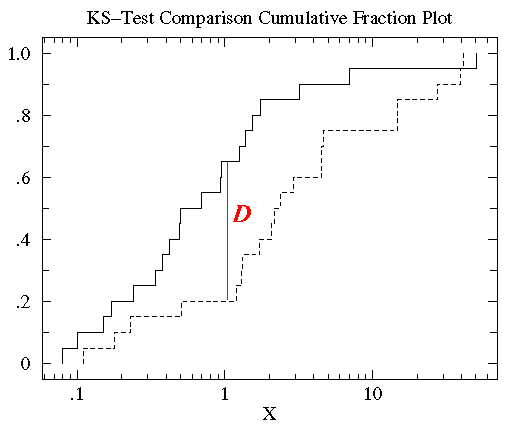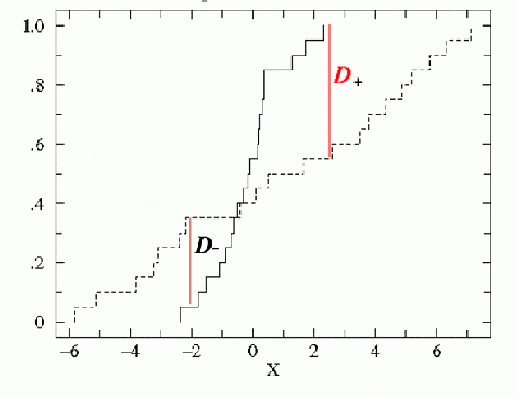## Summary

In the Chandra Source Catalog, a one-sample, two-sided Kolmogorov-Smirnov (K-S) test and a one-sample Kuiper's test are applied to the unbinned event data in each source region to measure the probability that the average intervals between arrival times of events are varying and therefore inconsistent with a constant source region flux throughout the observation. Corrections are made for good time intervals and for the source region dithering across regions of variable exposure during the observation. Note that background region information is not directly used in the K-S and Kuiper's variability tests in the Chandra Source Catalog, but is used in creating the Gregory-Loredo light curve for the background. The results of the K-S and Kuiper's variability tests are recorded in the columns ks_prob / kp_prob and ks_intra_prob / kp_intra_prob in the Source Observations Table and Master Sources Table, respectively.

#### Dither correction

One of the ways by which telescope dither introduces variability into light curves is via modulation of the fractional area of a source region as it moves as a function of time over a chip edge/boundary, or as it moves as a function of time to chip regions with differing numbers of bad pixels or columns. The fractional area (including chip edge, bad pixel, and bad column effects) vs. time curves for source regions are calculated from the data, and are sufficient to correct the K-S and Kuiper's tests used in the Chandra Source Catalog for the effects of dither. This correction is implemented in the K-S/Kuiper's test model by integrating the product of the good time intervals with the fractional area vs. time curve; the cumulative integral of this product is the cumulative distribution function against which the data is compared. For further details, see the memo "Adding Dither Corrections to L3 Lightcurves."

Note that the dither correction described above is a geometrical area correction only that is applied to the data; it does not take into account any spatial dependence of the chip responses. For example, if a soft X-ray source dithers from a frontside-illuminated chip to a backside-illuminated chip, the different soft X-ray responses of the two chips could introduce a dither period-dependent modulation of the detected counts that is not accounted for simply by geometrical area changes. The current catalog procedures do not correct for such a possibility; however, warning flags are set if sources dither across chip edges, and a dither warning flag is set if the variability occurs at the harmonic of a dither frequency.

## Background

### Kolmogorov-Smirnov Test

The K-S test is a goodness-of-fit test used to assess the uniformity of a set of data distributions. It was designed in response to the shortcomings of the chi-squared test, which produces precise results for discrete, binned distributions only. The K-S test has the advantage of making no assumption about the binning of the data sets to be compared, removing the arbitrary nature and loss of information that accompanies the process of bin selection.

In statistics, the K-S test is the accepted test for measuring differences between continuous data sets (unbinned data distributions) that are a function of a single variable. This difference measure, the K-S D statistic, is defined as the maximum value of the absolute difference between two cumulative distribution functions. The one-sided K-S test is used to compare a data set to a known cumulative distribution function, while the two-sided K-S test compares two different data sets. Each set of data gives a different cumulative distribution function, and its significance resides in its relation to the probability distribution from which the data set is drawn: the probability distribution function for a single independent variable x is a function that assigns a probability to each value of x. The probability assumed by the specific value xi is the value of the probability distribution function at xi and is denoted P(xi).The cumulative distribution function is defined as the function giving the fraction of data points to the left of a given value xi , P(x < xi) ; it represents the probability that x is less than or equal to a specific value xi.

Thus, for comparing two different cumulative distribution functions SN1(x) and SN2(x), the K-S statistic is

where SN(x) is the cumulative distribution function of the probability distribution from which a dataset with N events is drawn. If N ordered events are located at data points xi , i = 1, ... , N, then

where the x data array is sorted in increasing order. This is a step function that increases by 1/ N at the value of each ordered data point.###### Kirkman, T.W. (1996) Statistists to Use. http://www.physics.csbsju.edu/stats/

Though different data sets yield different cumulative distribution functions, all cumulative distribution functions agree at the smallest and largest allowable values of x (where they are zero and unity, respectively). Given that, it is clear why the K-S statistic is useful: it provides an unbiased measure of the behavior between the endpoints of multiple distributions, where they can be distinguished.

### Kuiper's Test

While the K-S test is adept at finding shifts in a probability distribution, with the highest sensitivity around the median value, its power must be enhanced by other techniques to be as good at finding spreads, which affect the tails of a probability distribution more than the median value. One such technique is Kuiper's test, which compares two cumulative distribution functions via the Kuiper's statistic V, the sum of the maximum distance of SN1(x) above and below SN2(x):###### Kirkman, T.W. (1996) Statistists to Use. http://www.physics.csbsju.edu/stats/

If one changes the starting point of the integration of the two probability distributions, D+ and D-change individually, but their sum is always constant. This general symmetry guarantees equal sensitivites at all values of x.